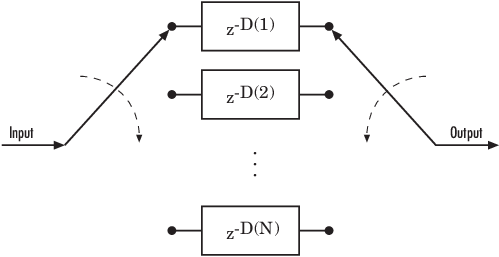# convdeintrlv

Restore ordering of symbols using shift registers

## Syntax

```deintrlved = convdeintrlv(data,nrows,slope) [deintrlved,state] = convdeintrlv(data,nrows,slope) [deintrlved,state] = convdeintrlv(data,nrows,slope,init_state) ```

## Description

`deintrlved = convdeintrlv(data,nrows,slope)` restores the ordering of elements in `data` by using a set of `nrows` internal shift registers. `slope` is the register length step. For information about delays, see Delays of Convolutional Interleaving and Deinterleaving.

`[deintrlved,state] = convdeintrlv(data,nrows,slope)` returns a structure that holds the final state of the shift registers. `state.value` stores any unshifted symbols. `state.index` is the index of the next register to be shifted.

`[deintrlved,state] = convdeintrlv(data,nrows,slope,init_state)` initializes the shift registers with the symbols contained in `init_state.value` and directs the first input symbol to the shift register referenced by `init_state.index`. The structure `init_state` is typically the `state` output from a previous call to this same function, and is unrelated to the corresponding interleaver.

### Using an Interleaver-Deinterleaver Pair

To use this function as an inverse of the `convintrlv` function, use the same `nrows` and `slope` inputs in both functions. In that case, the two functions are inverses in the sense that applying `convintrlv` followed by `convdeintrlv` leaves data unchanged, after you take their combined delay of `nrows*(nrows-1)*slope` into account. For information about delays, see Delays of Convolutional Interleaving and Deinterleaving.

## Examples

The example in Effect of Delays on Recovery of Convolutionally Interleaved Data Using MATLAB uses `convdeintrlv` and illustrates how you can handle the delay of the interleaver/deinterleaver pair when recovering data.

The example on the reference page for `muxdeintrlv` illustrates how to use the `state` output and `init_state` input with that function; the process is analogous for this function.

collapse all

### Delays of Convolutional Interleaving and Deinterleaving

The total delay due to a convolutional interleaver and deinterleaver pair is N × slope × (N – 1).

• N is the number of registers and equals the value of the `nrows` argument

• slope is the register length step and equals the value of the `slope` argument

This diagram shows the structure of a general convolutional interleaver comprised of a set of shift registers, each having a specified delay shown as D(1), D(2),..., D(N), and a commutator to switch input and output symbols through registers. The kth shift register holds D(k) symbols, where k = 1, 2, 3, … N. The kth shift register has a delay value of ((k–1) × slope). With each new input symbol, the commutator switches to a new register and shifts in the new symbol while shifting out the oldest symbol in that register. When the commutator reaches the Nth register, upon the next new input, the commutator returns to the first register.## References

 Heegard, Chris and Stephen B. Wicker. Turbo Coding. Boston: Kluwer Academic Publishers, 1999.

## Version History

Introduced before R2006a